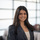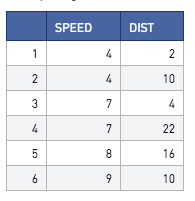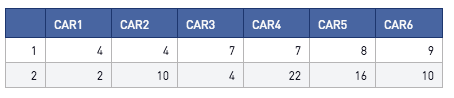# Transposing Tables in R

Transposing a dataframe in R is as simple as 2 quick lines of code. The first is to actually transpose the table, and the second is to name your column headers.

Below is the dataframe we will be transposing. This is pulled from using the head() command on the build in cars datasetThe R snippet below transposes the data. The last line, periscope.output(df2) passes the dataframe back into Periscope Data so you can use the built-in charting options.

```library(data.table)

#Transpose dataframe. Pass through df2 into periscope.table() to view transposed table
df2 = transpose(df)
colnames(df2) <- c("car1", "car2", "car3", "car4","car5", "car6")

periscope.table(df2)```

Yielding the result below:Prefer Python? Check out the community post here!